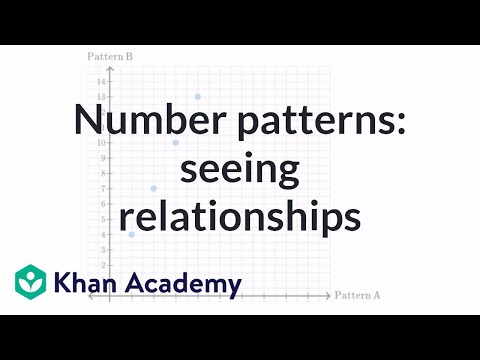Video

# Interpreting patterns on coordinate plane (Full video)

Description: Sal examines the points on a number line and interprets the patterns to discover relationships. Created by Sal Khan. So here, for each point, this point right over here, this represents its horizontal coordinate is the first term of pattern A, which is 4. And its vertical coordinate is the first term in pattern B, which is 1. Now, before even looking at these, let's see what we can think about these patterns here.

### Other videos you might be interested in### Operations and Algebraic Thinking

#### The Hunt Institute